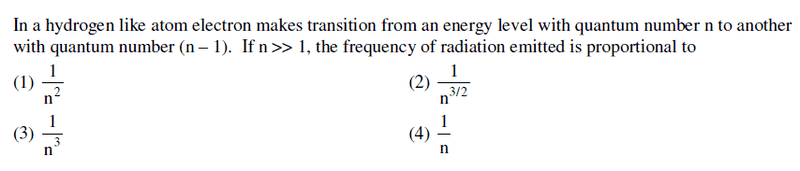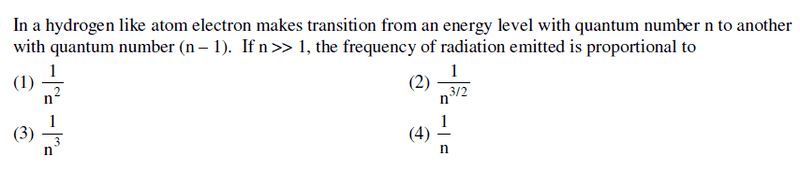# Approximation: The right way to do it

• Vibhor
In summary: I offered the following: "In summary, when making approximations, it is important to consider the purpose and level of accuracy needed. In some cases, it may be necessary to keep higher order terms, while in others, only the zeroth or first order terms are needed. It is important to carefully consider and understand the problem at hand before making any approximations."

## Homework StatementΔE = hf

## The Attempt at a Solution

f = ΔE/h = ##k\left [ \frac{1}{(n-1)^2} - \frac{1}{n^2} \right ]##

= ## \frac{k(2n-1)}{n^2(n-1)^2}##

= ## \frac{k}{n^4}(2n-1)(1-\frac{1}{n})^{-2}##

= ## \frac{2kn}{n^4}(1-\frac{1}{2n})(1+\frac{2}{n} +## higher powers ## )##

≈ ## \frac{2k}{n^3}(1-\frac{3}{2n}) ## . If I neglect the second term in the bracket , then I get option 3) , but I doubt whether I can do that because I need to retain atleast first order terms .

how should I proceed ?

Any help is appreciated .

Thanks

#### Attachments

Last edited:
•Delta2
Why do you think you need to retain the -3/2n term (which, incidentally, is wrong)?

It should be ≈ ## \frac{2k}{n^3}(1+\frac{3}{2n}) ## . Don't we retain the first order terms while making approximations ?

Vibhor said:
It should be ≈ ## \frac{2k}{n^3}(1+\frac{3}{2n}) ## . Don't we retain the first order terms while making approximations ?
It depends on the approximation. You keep as many terms as necessary to get an answer that is sufficiently accurate for purpose.
In many cases, e.g. in developing differential calculus, the zeroth order terms cancel, so it is essential to keep first order terms. In the present problem, the zeroth order term is enough.

•Vibhor
Ok .
haruspex said:
In many cases, e.g. in developing differential calculus, the zeroth order terms cancel, so it is essential to keep first order terms. In the present problem, the zeroth order term is enough.

Could you please explain with examples , at least twoVibhor said:
Ok .

Could you please explain with examples , at least twoLimit problems illustrate it. What is limit of (1-cos(x))x-2 as x tends to 0? When you expand the cos(x) as a power series, you have to keep the x2 term, but no further. But if you wanted the limit of (1-cos(x))x-1 you would only need to keep the zeroth and first order terms. Sometimes you find you did not keep enough and have to start again.

In a thread I recently got in an argument over, people who should have known better (including the problem setter) were effectively keeping some second order terms and discarding others. That's a no-no. It can lead you into thinking you have a valid solution when you do not.

•Vibhor and Delta2
haruspex said:
What is limit of (1-cos(x))x-2 as x tends to 0? When you expand the cos(x) as a power series, you have to keep the x2 term, but no further. But if you wanted the limit of (1-cos(x))x-1 you would only need to keep the zeroth and first order terms.

I think in both the cases we need to write cos(x) ≈ 1 - x2/2 .

What do you mean by "you would only need to keep the zeroth and first order terms " ?

Vibhor said:

## Homework StatementΔE = hf

## The Attempt at a Solution

f = ΔE/h = ##k\left [ \frac{1}{(n-1)^2} - \frac{1}{n^2} \right ]##

= ## \frac{k(2n-1)}{n^2(n-1)^2}##

= ## \frac{k}{n^4}(2n-1)(1-\frac{1}{n})^{-2}##

= ## \frac{2kn}{n^4}(1-\frac{1}{2n})(1+\frac{2}{n} +## higher powers ## )##

≈ ## \frac{2k}{n^3}(1-\frac{3}{2n}) ## . If I neglect the second term in the bracket , then I get option 3) , but I doubt whether I can do that because I need to retain atleast first order terms .

how should I proceed ?

Any help is appreciated .

Thanks
For large ##n>0## we have
$$\frac{1}{(n-1)^2} = \frac{1}{n^2} \left(1 - \frac{1}{n} \right)^{-2} = \frac{1}{n^2} \left( 1 + \frac{2}{n} + \cdots \right) \doteq \frac{1}{n^2} + \frac{2}{n^3}$$

Vibhor said:
I think in both the cases we need to write cos(x) ≈ 1 - x2/2 .

What do you mean by "you would only need to keep the zeroth and first order terms " ?
If we keep only the zeroth and first order terms of the cos(x) expansion in (1-cos(x))x-1 we get (1-1+(0)x+O(x2))x-1=0+O(x), which tends to 0.

Ray Vickson said:
For large ##n>0## we have
$$\frac{1}{(n-1)^2} = \frac{1}{n^2} \left(1 - \frac{1}{n} \right)^{-2} = \frac{1}{n^2} \left( 1 + \frac{2}{n} + \cdots \right) \doteq \frac{1}{n^2} + \frac{2}{n^3}$$

We have to multiply this by ## \frac{k(2n-1)}{n^2}##

## ≈ k \left ( \frac{2}{n^3} + \frac{3}{n^4} - \frac{2}{n^5}\right ) ##

Now , since ##\frac{2}{n^3}## is the biggest and the most dominant term , hence we neglect the other terms and keep only the ## \frac{2}{n^3}## .

Is this what you are trying to convey ?

Vibhor said:
We have to multiply this by ## \frac{k(2n-1)}{n^2}##

## ≈ k \left ( \frac{2}{n^3} + \frac{3}{n^4} - \frac{2}{n^5}\right ) ##

Now , since ##\frac{2}{n^3}## is the biggest and the most dominant term , hence we neglect the other terms and keep only the ## \frac{2}{n^3}## .

Is this what you are trying to convey ?

The way you are doing it requires the factor ##k(2n-1)/n^2##, but not the way I am doing it. Look again.

nrqed said:
I agree that the -3/2 n should be dropped but why are you saying it is wrong? The OP included all the terms of order 1/n in the parenthesis so it looks to me that the coefficient -3/2 is actually correct.
The sign is wrong. Vibhor corrected it in post #3.

Ray Vickson said:
The way you are doing it requires the factor ##k(2n-1)/n^2##, but not the way I am doing it. Look again.
Ray, I see no real difference between your method and Vibhor's. Isn't it just a matter of the order of the steps? If you take one more term in your expansion you will get Vibhor's original result (with the sign correction).

haruspex said:
Ray, I see no real difference between your method and Vibhor's. Isn't it just a matter of the order of the steps? If you take one more term in your expansion you will get Vibhor's original result (with the sign correction).

Well, I was hoping to get the OP to look at things more simply. It is a lot easier to get
$$f/k = \frac{1}{(n-1)^2} - \frac{1}{n^2}$$
directly as
$$f/k = \left( \frac{1}{n^2} + \frac{2}{n^3} + \frac{3}{n^4} + \cdots \right) - \frac{1}{n^2} \\ = \frac{2}{n^3} + \frac{3}{n^4} + \cdots$$
than by writing
$$f/k = \frac{2n-1}{n^2} \left( \frac{1}{n^2} + \frac{2}{n^3} + \frac{3}{n^4} + \cdots \right)$$
and then doing more algebra plus collecting terms, etc. The OP did ask for the right way to do it.

•Vibhor
haruspex said:
The sign is wrong. Vibhor corrected it in post #3.
Oops. Gotcha. I was so focused on checking the 3/2 that I did not pay attention to the sign. Sorry.